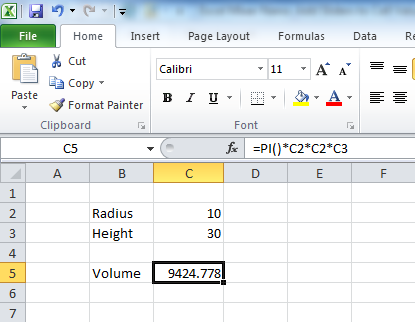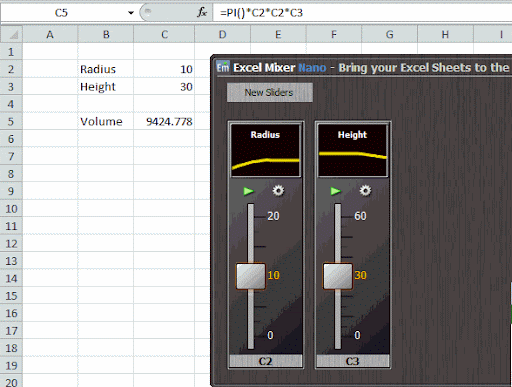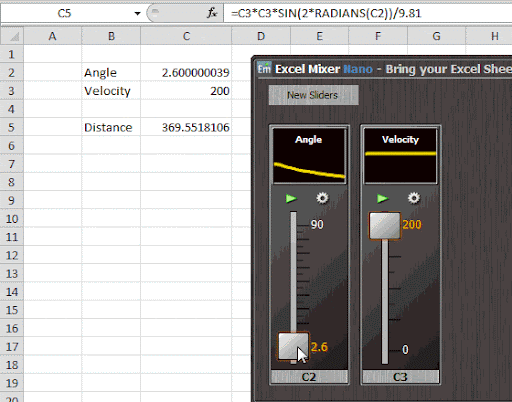# Instant Fundas

## Excel Mixer Nano: Add Sliders to Control Cell Value. See Results in Real-Time

>

Excel Mixer Nano is a fantastic add-on for Microsoft Excel that allows you to adjust values in a cell with the help of sliders and observe in real-time how the result of a formula change as you vary the inputs. It can be used to study how different inputs in a mathematical or scientific formula has an effect on the result.

To illustrate, here is an Excel sheet that calculates the volume of a cylinder from the radius and height of the cylinder. The volume of the cylinder is given by (Pi) x (radius)2 x (height).The values assigned to radius and height are currently 10 and 30. But I want to see how the volume of the cylinder would change if I change the radius and the height of the cylinder.

So I fire up Excel Mixer Nano, click on the value 10 and then click on the ‘New Slider’ button. This creates a slider that allows me to adjust the value of the selected cell. I do the same for height and get another slider. Now as I move both sliders up and down, the result in the formula cell changes in real-time too.In another practical example, I’m trying to find out how the distance of a projectile varies as you change the angle and velocity. Notice, the maximum distance is achieved at 45 degrees.Though I’m using only two variables in my examples, there is no limit to the number of variable cells you can use.

Excel Mixer Nano doesn’t actually integrate with Excel. It’s a standalone program that can read data from an Excel sheet and feed data back to it. It doesn’t even require installation because it’s a portable program.

The Pro version of this program is vastly more powerful that can produce 3-dimensional graph of the output, allowing one make a visual analysis of mathematical and engineering equations. Watch the video demo of Excel Mixer Pro.

#### 1 comment:

1.This is definitely one of the best websites i have EVER come across with tips and tricks. Keep up the great work!

Name

Email *

Message *